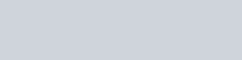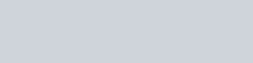Using integration, find the area of
Question:

Using integration, find the area of $\triangle \mathrm{ABC}$, the equation of whose sides $A B, B C$ and $A C$ are given by $Y=4 x+5, x+y=5$ and $4 y=x+5$ respectively.

Solution: